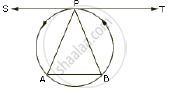Share

# P is the Mid – Point of an Arc Apb of a Circle. Prove that the Tangent Drawn at P Will Be Parallel to the Chord Ab. - Mathematics

Course

#### Question

P is the mid – point of an arc APB of a circle. Prove that the tangent drawn at P will be parallel  to the chord AB.

#### SolutionJoin AP and BP.
Since TPS is a tangent and PA is the chord of the circle.
∠BPT =  ∠PAB (angles in alternate segments)
But

∠PBA = ∠PAB (∵ PA = PB )

∴ ∠ BPT =∠PBA

But these are alternate angles

∴ TPS ll AB

Is there an error in this question or solution?